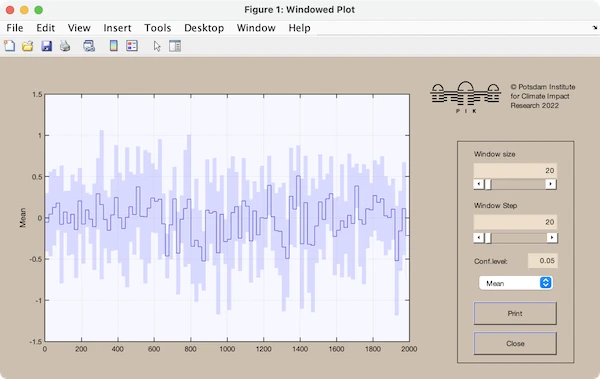# CRP Toolbox

## winplot

Windowed plot.

### Syntax

``````winplot(x)
winplot(x,w,ws)
winplot(x,w,ws,flag)
y=winplot(x,'parm')
``````

### Description

winplot(x [,w,ws]) plots means or variances of the sub-vectors of vector x, which have the length w and are shifted by the step ws. x can be a two-column vector, where the first column would be the time-scale.

winplot without any arguments calls a demo (the same as the example below).

### Parameters

The optional parameter flag can determine the kind of the result, where flag can be either a string or a scalar:
 'mean' or 1 - Mean (1st moment). 'var' or 2 - Variance (2nd moment). 'std' or 3 - Standard deviation. 'median' or 4 - Median. 'sqm' or 5 - Squared Mean. 'geo' or 6 - Geometric Mean. '3rd' or 7 - 3rd moment. 'skw' or 8 - Skewness. 'kur' or 9 - Kurtosis.

### Example

``````winplot(randn(2000,1),20,20)
``````